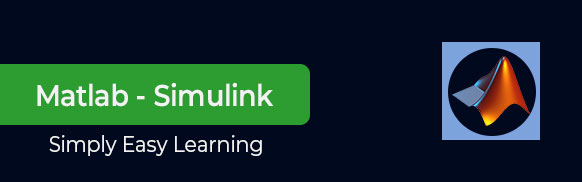MATLAB (Matrix Laboratory) is a programming language developed by a computer software company MathWorks. Simulink is a simulation and model-based design environment for dynamic and embedded systems, which are integrated with MATLAB. Simulink is also developed by MathWorks. This tutorial is designed to give students fluency in MATLAB Simulink. Problem-based examples have also been given in simple and easy way to make your learning fast and effective.

Audience

This tutorial has been prepared for the beginners to help them understand basic to advanced functionality of MATLAB Simulink. After completing this tutorial you will find yourself at a moderate level of expertise in using Simulink from where you can take yourself to next levels.

Prerequisites

We assume you have a little knowledge of any computer programming and understand concepts like variables, constants, expression, statements, etc. If you have done programming in any other high-level programming language like C, C++ or Java, then it will be very much beneficial and learning MATLAB Simulink will be like a fun for you.

Useful Video Courses

Video

Data Preprocessing for Machine Learning using MATLAB

30 Lectures 4 hours

Video

Complete MATLAB Tutorial: Go from Beginner to Expert

127 Lectures 12 hours

Video

Image Processing Toolbox in MATLAB

17 Lectures 3 hours

Video

Matlab - The Complete Course

37 Lectures 5 hours

Video

Digital Image Processing using MATLAB

22 Lectures 4 hours

Video

Backpropagation Learning Method in Matlab

18 Lectures 3 hours